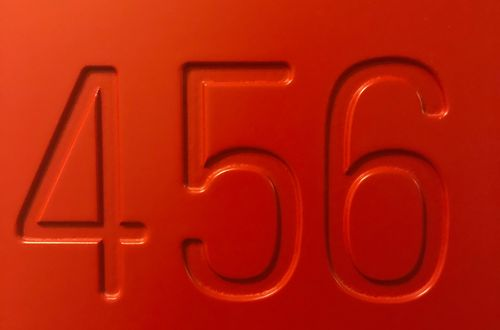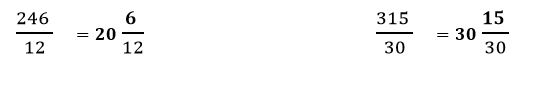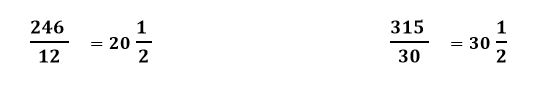# How to express mixed fractions as improper fractions

Want to find out how to express mixed fractions as improper fractions? We've provided a step-by-step guide of how to express mixed fractions as improper fractions to teach you everything you need to know.## Step 1: Multiply the number and the denominator

Multiply the whole number part and denominator and take note of the product. For example, to change 3 1/4 to an improper fraction, we multiply 3 and 4 first.

## Step 2: Add the product to the numerator

Next would be to add the product to the fraction part’s numerator.

## Step 3: Write down the sum as the numerator

We now express the mixed fraction as improper fraction by writing down the sum as the numerator.

## Examples of how to express mixed fractions as improper fractions

Q1) Express the following improper fractions as mixed fractions. a. 246/12 b. 315/30

To change the improper fractions to mixed fractions, we divide the numerator by the denominator.

Hence, we have: 246 /12 315/30 246÷12=20 R 6 315÷30=30 R 15

Quotient =20 Quotient =30 Remainder = 6 Remainder = 15

Once we have the quotient and remainder, we can write the quotient as the whole number part and the remainder as the numerator of the mixed fraction.Check if the fraction if simplified, if not, we can reduce them to lowers terms as well.#### Join today

The fastest way to practice

Unlock our complete testing platform and improve faster that ever.